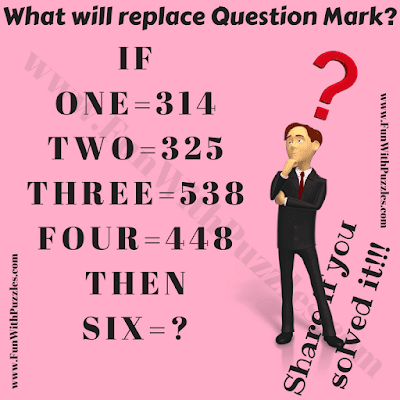## Thursday, June 25, 2020

Here is the logical reasoning puzzle question for kids and school going teens. Some basic knowledge of English is required to solve this logical reasoning puzzle. In this Puzzle Question, some English words are equated to numbers logically. Can you find this relationship between words and number and then find the value of missing number which will replace the question mark?Can you solve this logical reasoning puzzle question?

The answer to this "Logical Reasoning Puzzle Question", can be viewed by clicked on the button below.

1.1.2.369 is the answer because count the digits like one these are three digits second step see the number like six these are three digits third step add these both numbers answer will come 369😊😊😊

1.Yes, your answer and explanation to this logical reasoning puzzle question is right.

3.1.672 is not the right answer to this logical reasoning puzzle question. It will be great if you can post your logical reasoning for your answer.

4.369 is answer it is correct

5.369...1st we have to count the letters in the given word 2nd we have to see the order of number like 1,2,3...then 3rd we Have to add 1st and 2nd ...Number ,hence u will get ur answer...

6.Why 369? Why not 167 or 268?

1.